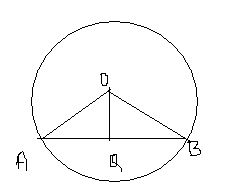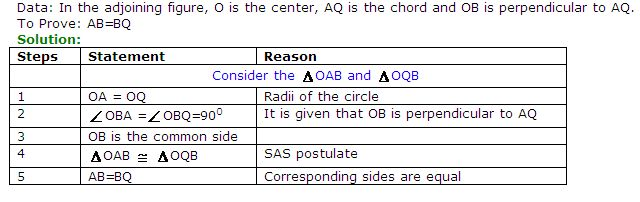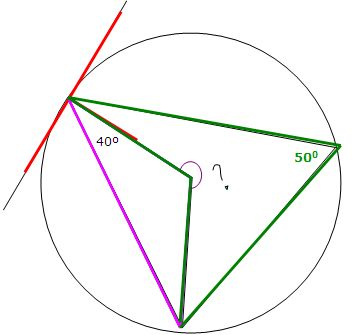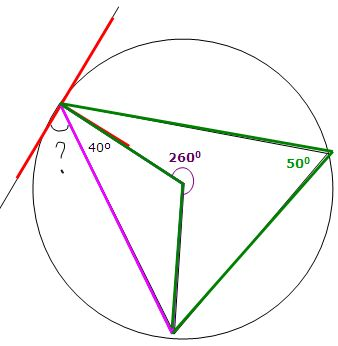kwizNET Subscribers, please login to turn off the Ads!
 Email us to get an instant 20% discount on highly effective K-12 Math & English kwizNET Programs!

#### Online Quiz (WorksheetABCD)

Questions Per Quiz = 2 4 6 8 10

### High School Mathematics - 28.7 Circles

 Radius: A line joining the centre to any point on the circle. Diameter: Two times the radius is the diameter. Chord: A line segment joining any two points on the circle is chord. Major Arc and Minor Arc: Any two points A and B of a circle divide the circle into two points. If the two parts are unequal, the smaller part is called minor arc and the larger part is called major arc. A circle divides the plane into three parts, its interior exterior and the circle itself. The circle along with its interior is called the circular region. A chord divides a circular region into two parts called the segments of the region. The smaller segment is called the minor segment and the larger segment is called the major segment Theorem 1: In a circle, the perpendicular from the center to the chord bisects the chord.Theorem 2: Chords of a circle which are equidistant from the centre are equal.Given: OM = ON (radii of the circle) To prove: AB = CD Construction: Join AO and CO Proof: Consider triangles AMO and CNO OM = ON Angle M = Angle N = 90o Angle AOM = CON (common angle) Hence triangles AMO and CNO are similar. Hence AB = CD Directions: Solve the following.
 Q 1: AB and CD are two parallel chords of a circle lying on the opposite side of the centre. AB = 10 cm and CD = 24 cm. If the distance between the chords is 17 cm, find the radius of the circle.Answer: Q 2: Find the length of the chord if the radius of circle is 13 cm and distance from the centre is 5 cm.Answer: Q 3: PQ and RS are two parallel chords of a circle whose radius is 10 cm. If PQ = 16 cm and RS = 12 cm, find the distance between the chords if they lie on the same side of the centre.Answer: Q 4: Find the missing angle.150 degrees260 degrees100 degrees Q 5: Find the missing angle.75 degrees50 degrees110 degrees Q 6: Find the missing angle.Both are 50 degreesBoth angles are 40 degrees.Both angles are 35 degrees. Q 7: PQ and RS are two parallel chords of a circle whose radius is 10 cm. If PQ = 16 cm and RS = 12 cm, find the distance between the chords if they lie on the opposite side of the centre.Answer: Q 8: AB and CD are two equal chords to a circle of centre O. If OM is perpendicular to AB and ON is perpendicular to CD. Prove that angle OMN = angle ONMAnswer: Question 9: This question is available to subscribers only! Question 10: This question is available to subscribers only!

#### Subscription to kwizNET Learning System offers the following benefits:

• Unrestricted access to grade appropriate lessons, quizzes, & printable worksheets
• Instant scoring of online quizzes
• Progress tracking and award certificates to keep your student motivated
• Unlimited practice with auto-generated 'WIZ MATH' quizzes
• Child-friendly website with no advertisements
• Choice of Math, English, Science, & Social Studies Curriculums
• Excellent value for K-12 and ACT, SAT, & TOEFL Test Preparation
• Get discount offers by sending an email to discounts@kwiznet.com

 Quiz Timer